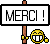[Livre] A First Course in Random Matrix Theory - For physicists, engineers and data scientists
A First Course in Random Matrix Theory
For physicists, engineers and data scientists

The real world is perceived and broken down as data, models and algorithms in the eyes of physicists and engineers. Data is noisy by nature and classical statistical tools have so far been successful in dealing with relatively smaller levels of randomness. The recent emergence of Big Data and the required computing power to analyse them have rendered classical tools outdated and insufficient. Tools such as random matrix theory and the study of large sample covariance matrices can efficiently process these big data sets and help make sense of modern, deep learning algorithms. Presenting an introductory calculus course for random matrices, the book focusses on modern concepts in matrix theory, generalising the standard concept of probabilistic independence to non-commuting random variables. Concretely worked out examples and applications to financial engineering and portfolio construction make this unique book an essential tool for physicists, engineers, data analysts, and economists.

[Lire la suite]